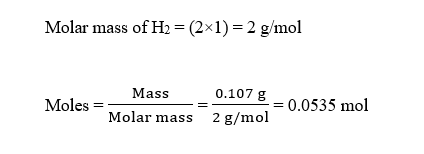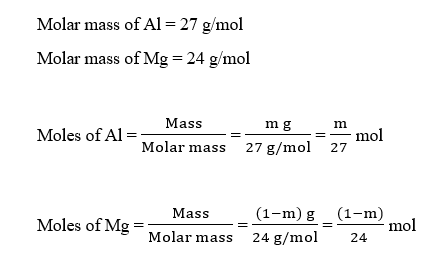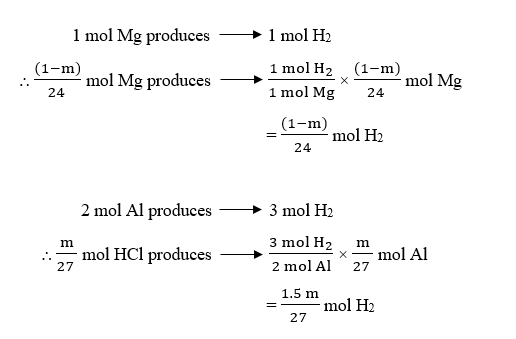# A 1.00 g sample of a magnesium-aluminum alloy yields 0.107 g H2 when treated with an excess of HCl(aq). What is the percentage by mass of Al in the alloy? [Hint: This is the inverse of Example 4-16. To solve this problem, let m and 1.00 - m be the masses of Al and Mg, respectively, and then use these masses in the setup above to develop an equation that relates m to the total mass of H2 obtained. Then solve for m.]

Question
15 views

A 1.00 g sample of a magnesium-aluminum alloy yields 0.107 g H2 when treated with an excess of HCl(aq). What is the percentage by mass of Al in the alloy? [Hint: This is the inverse of Example 4-16. To solve this problem, let m and 1.00 - m be the masses of Al and Mg, respectively, and then use these masses in the setup above to develop an equation that relates m to the total mass of H2 obtained. Then solve for m.]

check_circle

Step 1

Given,

Mass of Mg-Al alloy = 1.00 g

Mass of H2 produced = 0.107 g

Moles of H2 produced can be calculated as :Step 2

Let the mass of Al be ‘m’ g, then the mass of Mg will be (1.00 – m) g.

The moles of Al and Mg will be :Step 3

The balanced reaction of Mg and Al with HCl can be written as:

Mg (s) + 2 H⁺ (aq) → Mg²⁺ (aq) + H₂ (g)

2 Al (s) + 6 H⁺ (aq) → 2Al³⁺(aq) + 3 H₂(g)

From the balanced reaction, it is evident that :...

### Want to see the full answer?

See Solution

#### Want to see this answer and more?

Solutions are written by subject experts who are available 24/7. Questions are typically answered within 1 hour.*

See Solution
*Response times may vary by subject and question.
Tagged in